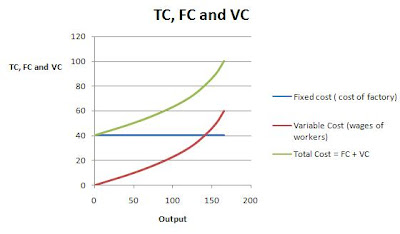### Total Cost, Fixed Cost and Variable Cost curves

Fixed Cost - is the cost of fixed factors of production. Fixed Cost remains the same in the short run.

Variable Cost - is the cost of variable factors of production. Variable Cost increases with the increase in the quantity of production.

TC = TFC + TVC

(Total cost = Total Fixed Cost + Total variable Cost)

Consider a factory where factory is a fixed factor and the workers are variable factor.

 Workers ( variable factor) Output Fixed cost ( cost of factory) Variable Cost (wages of workers) Total Cost = FC + VC 0 0 40 0 40 1 50 40 10 50 2 90 40 20 60 3 120 40 30 70 4 140 40 40 80 5 155 40 50 90 6 165 40 60 100

Drawing these points on a graph, the curves will be -· Fixed cost remains the same at all levels of output.

· As the output increases, TC and Variable Cost increases.

· TC = FC + VC